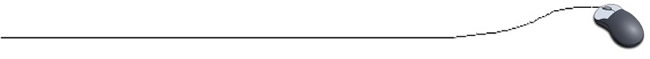The "if" Statement It's time to make decisions using the relational operators.

The "if" expression is called a conditional statement (decision statement) because it tests a relationship by using the relational operators.  Based upon the result of the comparison, decisions are made as to which statement(s) the program will execute next.

 if (test condition)   {       block of one;       or more Java statements; }

The test condition can be any relational comparison (or logically TRUE) statement and must be enclosed in parentheses.  The block of statements is enclosed in braces and indented for readability.

• The braces are NOT required if only ONE statement follows the "if", but it is a good idea to get in the habit of including them.

• If you declare a variable inside the block, the variable has block scope, meaning it can only be used within the block. The rest of the program will not recognize that variable.If you put a semicolon after the test condition, the "if" statement will STOP.  No condition will be checked. It will be as if the line was never in the program. The block, however, WILL be executed as the next available line of code.

The block executes only if the test condition is TRUE.  If the test condition is FALSE, the block is ignored and execution continues to the next statement following the block.

 Strange, but true!!! if (a = 3)   will cause an ERROR.  The condition a = 3 is an assignment statement. It is assigning 3 to the variable a. It is not checking to see if a contains a 3. "Test conditions" are looking for the later, for Boolean true or false expressions. if (a = = 3)  is a boolean test condition.  This statement is true ONLY if the value stored in a is the number 3. Remember, computers do not interpret equality in the same way that we do in mathematics.

Examples:

 // using a character literal if (grade = = 'A') {    System.out.println("Fridge Grade"); } // logically true if (true) {     System.out.println("Way to go!");     System.out.print("The x is true!"); } // using two variables if (enemyCount < heroCount) {     System.out.print("Good wins!"); } // using a calculation if (cost * number  = = paycheck) {     inventory = 0; }

Boolean:
A boolean value is one that is either true or false.
Be careful: You may have seen 1's and 0's being used as "true" or "false" (perhaps on your calculator, or in C++). Java does NOT recognize "1" or "0" to represent "true" or "false".

 boolean value = true; if (value == true) {    System.out.println("true"); } else {    System.out.println("false"); } Since "value" was declared to be "true", this code will print "true".

Alternate codes:

 Check out this alternate way to produce the same result as shown above. boolean value = true; if (value) {    System.out.println("true"); } else {    System.out.println("false"); } Prints "true" In this example, the ! symbol, which means "not", is used. boolean value = true; if (!value) {    System.out.println("true"); } else {    System.out.println("false"); } Prints "false"